SSOJ2551地铁费用
693+

题目描述

（注意：不能到达的城市，不需要任何费用，即费用为0；两个城市之间可以有多条直接相连的地铁，且费用不一样。）

n行，第i行表示到城市i的费用。

6 5 1
1 2 5
2 3 8
1 4 2
4 5 2
5 3 2

0
5
6
2
4
0

提示

5 <= n <= 10000
10 <= m <= 100000
1 <= a、b <= n
1 <= c <= 100
60%的数据：n <= 5000
80%的数据：n <= 8000

程序实现

60分程序，开5000*5000数组，不会爆内存：80分程序，开8000*8000的short数组，也不会爆内存：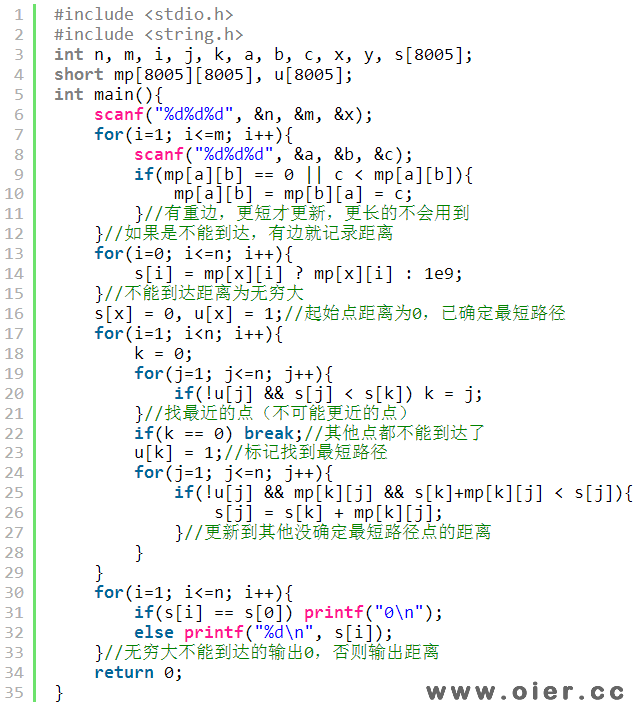100分迪杰斯特拉程序，开10000*10000的char数组，不会爆内存，要想不超时，第25行的条件需要注意了：(mp[k][j] && s[k]+mp[k][j] < s[j])不会超时，如果写成(!u[j] && mp[k][j] && s[k]+mp[k][j] < s[j])或者(s[k]+mp[k][j] < s[j] && mp[k][j])则有可能过不了第10个点，为什么？想想就知道了！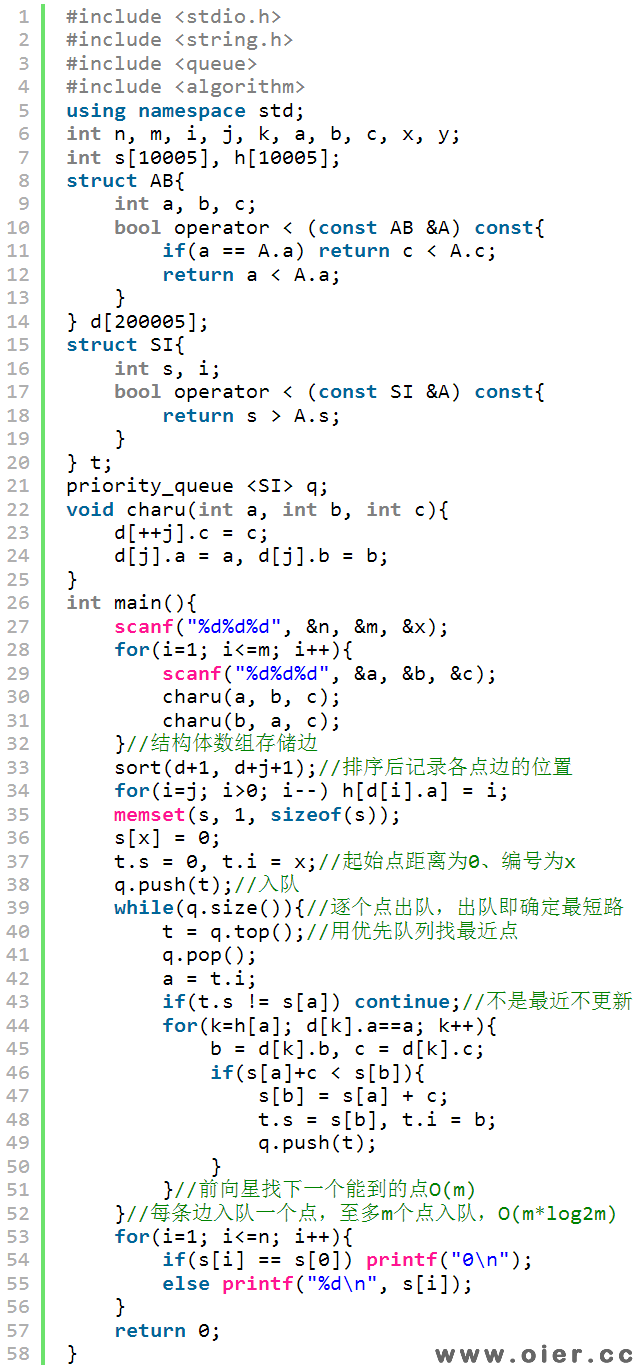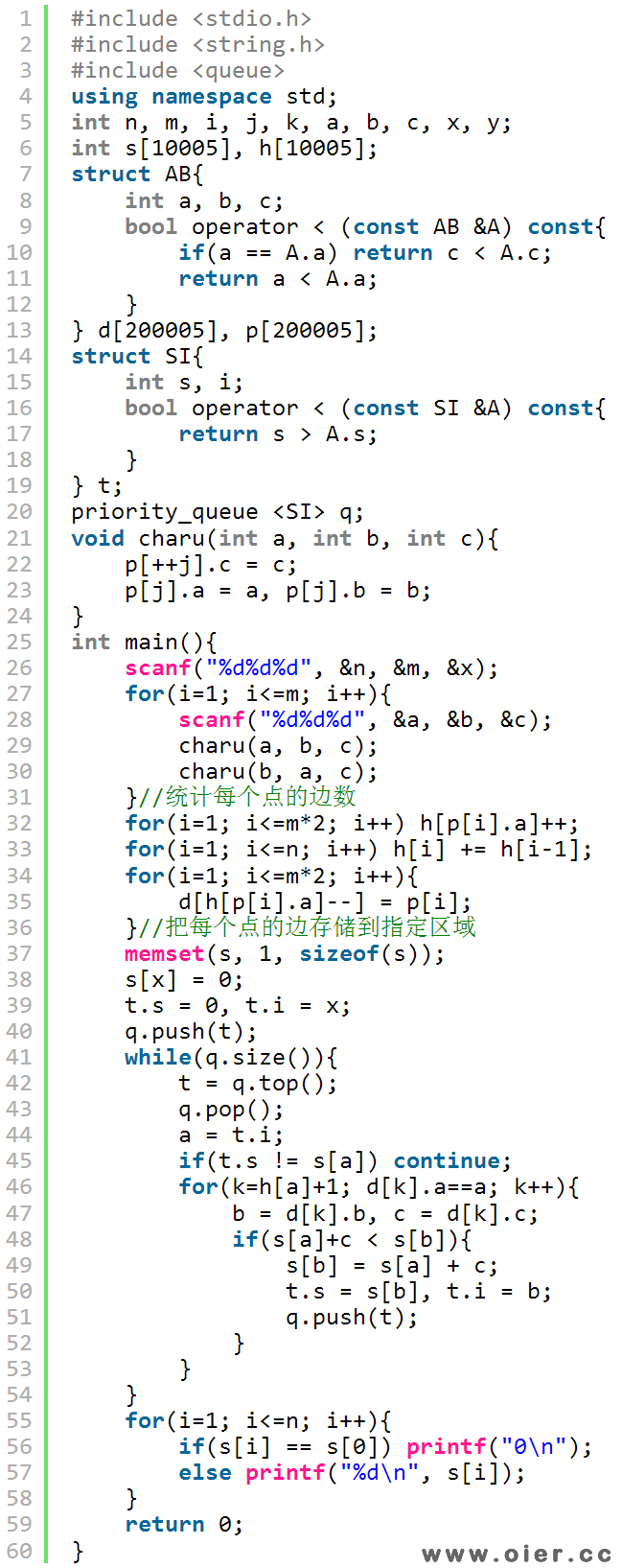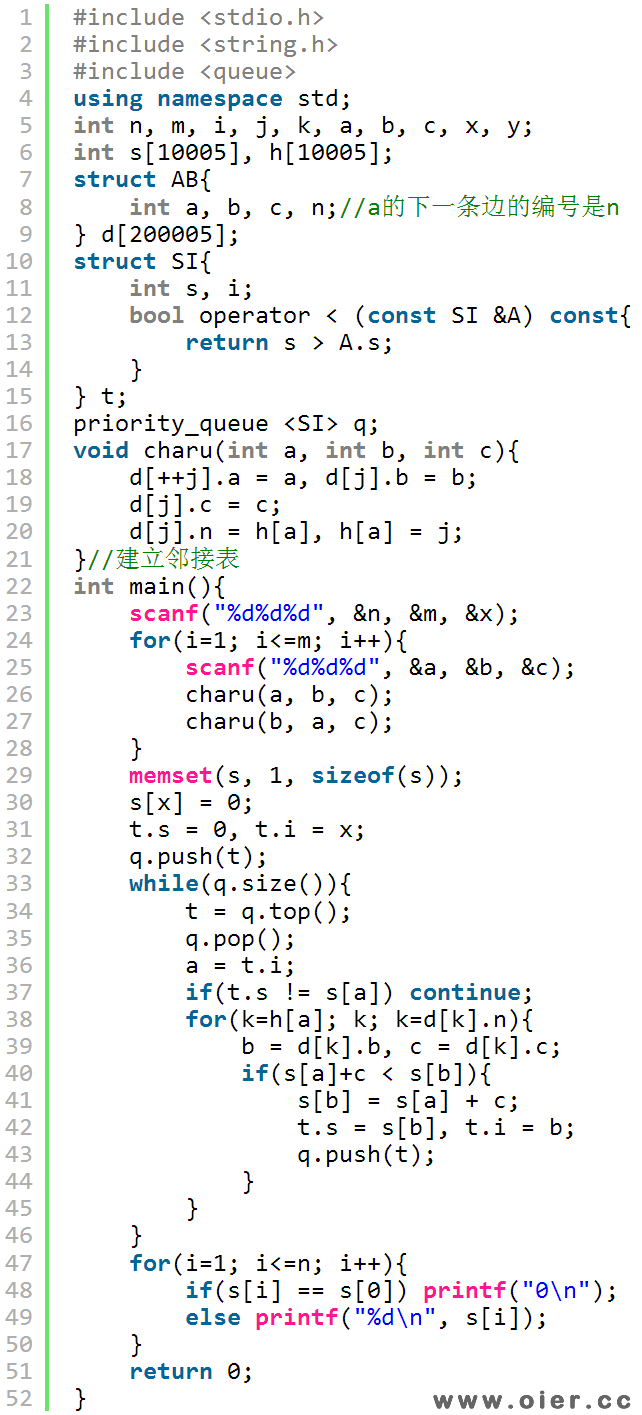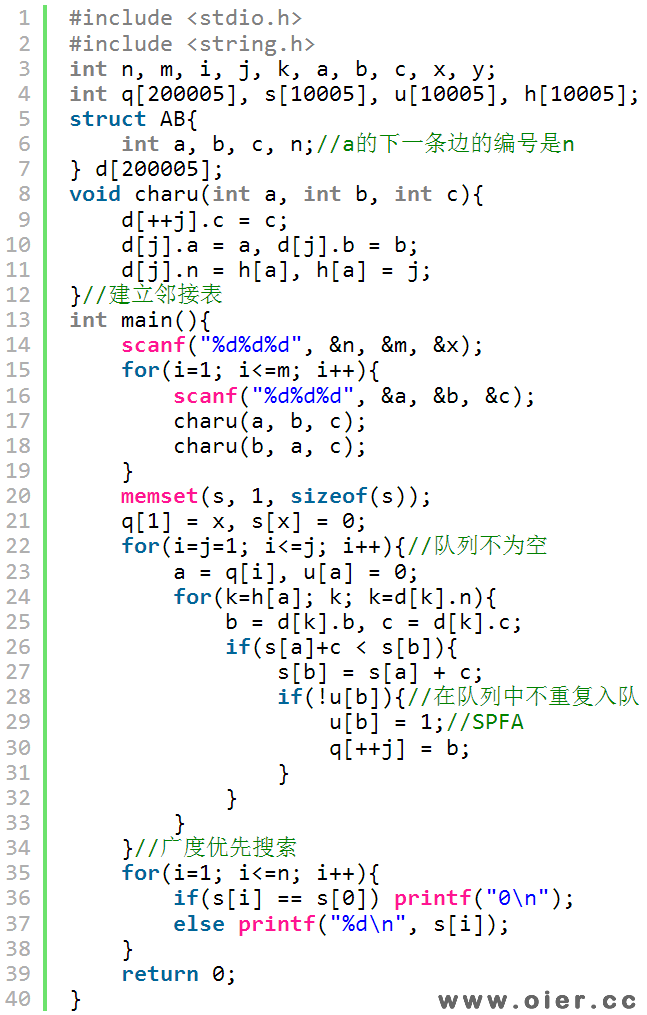SSOJ2551地铁费用：等您坐沙发呢！